# Evaluating Expressions

##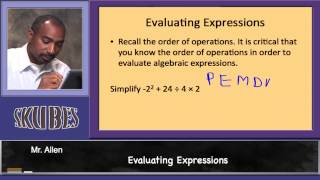By Skubes Ed

Interpret expressions that represent a quantity in terms of its context. Interpret parts of an expression, such as terms, factors, and coefficients.# Evaluating Expressions with Two Variables - Khan Academy

##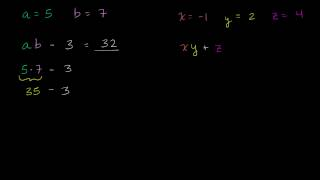By Khan Academy

Sal Khan shows the viewer how to evaluate an expression with two variables.# Examples: Writing Variable Expressions

##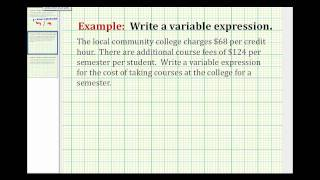By Mathispower4u

Practicing variable expressions with this tutorial video.# Evaluating Expressions with Parentheses and Braces and Brackets

##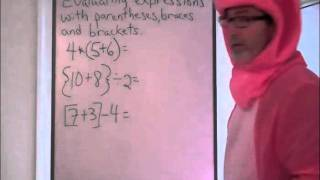By HoodaMathTutorials

The instructor dressed as a pig shows how to solve expressions using parentheses braces and brackets. He explains that when these symbols are used they mean to "do me first" in order of operations.# Evaluating Expressions Involving Absolute Value - Example 1

##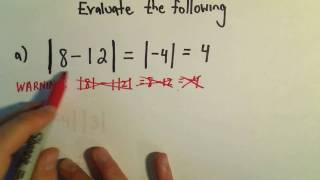By PatrickJMT

This video explains how to evaluate expressions involving absolute value. The examples include a few very basic problems involving simplifications with absolute value.# Evaluating Expressions with Parentheses

##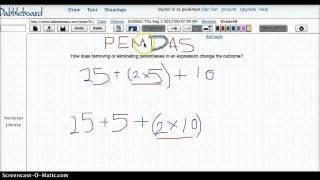By KFreimuth83

This Screencast was completed using Dabbleboard and shows how changing removing or eliminating parentheses with similar numbers can change the outcome. The instructor reviews the order of operations as she works the same problem in two different ways based on placement of parentheses.# Evaluating expressions with variables word problems

##By Khan Academy

Learn how to evaluate an expression with variables using a technique called substitution (or plugging in).# Evaluating expressions with variables word problems

##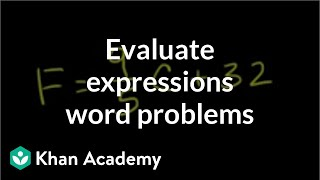By Khan Academy

In this example we have a formula for converting Celsius temperature to Fahrenheit. Let's substitute the variable with a value (Celsius temp) to get the degrees in Fahrenheit. Great problem to practice with us!# Evaluating expressions with variables word problems

##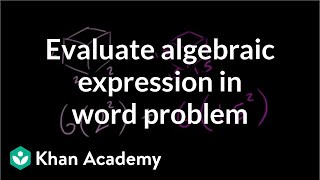By Khan Academy

In this example of evaluating expressions, we're dusting off some geometry. On top of that, it's a word problem. We're seeing how different concepts in math are layered on top of each to create more interesting and complex problems to solve.# Algebraic Expressions (Basics)

##By Anywhere Math# Trigonometric Substitution

##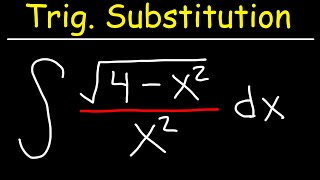By The Organic Chemistry Tutor

This calculus video tutorial provides a basic introduction into trigonometric substitution. It explains when to substitute x with sin, cos, or sec. It also explains how to perform a change of variables using u-substitution integration techniques and how to use right triangle trigonometry with sohcahtoa to convert back from angles in the form of theta to an x variable. There's plenty of examples and practice problems in this lesson.##By Anywhere Math# ALL OF GRADE 9 MATH IN 60 MINUTES!!! (exam review part 1)

##By Lumos Learning

Here is a great exam review video reviewing all of the main concepts you would have learned in the MPM1D grade 9 academic math course. The video is divided in to 3 parts. This is part 1: Algebra. The main topics in this section are exponent laws, polynomials, distributive property, and solving first degree equations. Please watch part 2 and 3 for a review of linear relations and geometry. If you watch all 3 parts, you will have reviewed all of grade 9 math in 60 minutes. Enjoy! Visit jensenmath.ca for more videos and course materials.# 05 - Simplify Irrational Exponents, Part 1 (Radical Exponents, Powers, Pi & More)

##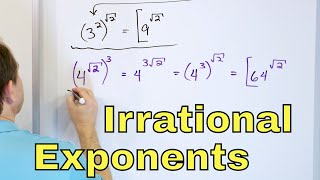By Math and Science

Quality Math And Science Videos that feature step-by-step example problems!